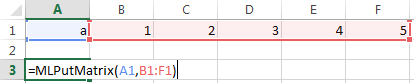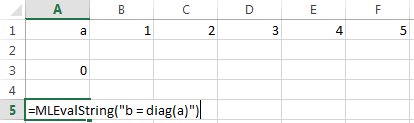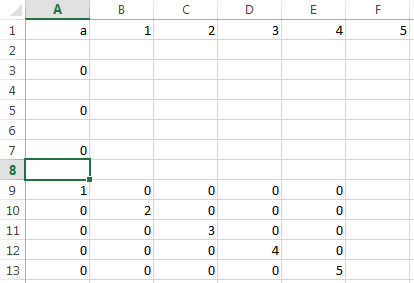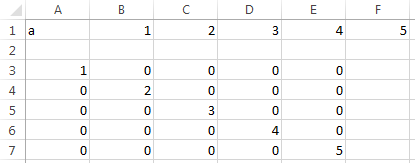# MLEvalString

Evaluate MATLAB command in MATLAB

## Syntax

``= MLEvalString(command)``
``MLEvalString command``
``err = MLEvalString(command)``

## Description

example

````= MLEvalString(command)` specifies the MATLAB® command for evaluation in the MATLAB workspace. Use this syntax when working in a worksheet cell.```

example

````MLEvalString command` works in a VBA macro.```

example

````err = MLEvalString(command)` returns the execution status when executing `MLEvalString` in a VBA macro.```

## Examples

collapse all

Enter the variable `a` into cell `A1`. Enter the numbers 1 through 5 into the range of cells from `B1` through `F1`.

Assign the range of cells to variable `a` in MATLAB using `MLPutMatrix`. Enter this text in cell `A3`.

`=MLPutMatrix(A1,B1:F1)`Use `diag` to create a matrix `b`, containing a diagonal using the five numbers in variable `a`. Enter this text in cell `A5`.

`=MLEvalString("b = diag(a);")`Retrieve matrix `b` from MATLAB into Excel®, cell `A9`. Enter this text in cell `A7`.

`=MLGetMatrix("b","A9")`

The matrix with the diagonal appears in cells `A9` through `E13`.Enter the variable `a` into cell `A1`. Enter the numbers 1 through 5 into the range of cells from `B1` through `F1`.

Click the Developer tab in Microsoft® Excel, and then click . The Visual Basic® Editor window opens.

Insert a new module and enter this VBA code into the Code section of the window. This sample code assumes a macro named `Diagonal`. For details on working with modules, see Excel Help.

```Sub Diagonal() MLPutMatrix "a", Range("B1:F1") MLEvalString "b = diag(a);" MLGetMatrix "b", "A3" MatlabRequest End Sub```

Run the macro by clicking . The diagonal matrix appears in cells `A3` through `E7`. For details on running macros, see Excel Help.Enter the variable `a` into cell `A1`. Enter the numbers 1 through 5 into the range of cells from `B1` through `F1`.

Click the Developer tab in Microsoft Excel, and then click . The Visual Basic Editor window opens.

Insert a new module and enter this invalid VBA code into the Code section of the window. This sample code assumes a macro named `Diagonal`. For details on working with modules, see Excel Help.

```Sub Diagonal() Dim err As Variant MLPutMatrix "a", Range("B1:F1") err = MLEvalString("b = diag(2a);") 'Invalid code If err <> 0 Then MsgBox err End If MLGetMatrix "b", "A3" MatlabRequest End Sub```

Run the macro by clicking . For details on running macros, see Excel Help.

This Spreadsheet Link™ error displays: `#COMMAND!`. To display MATLAB errors, see `MLShowMatlabErrors`.

## Input Arguments

collapse all

MATLAB command to evaluate, specified as a string. Enclose the string in double quotes. Or, enter the string in a cell without quotes and enter the corresponding cell reference without quotes as the input argument.

Example: `"sum"`

Example: `A1`

## Output Arguments

collapse all

Execution status, returned as a string or number. If `MLEvalString` fails, then `err` is a string containing an error code or error message. Otherwise, the command executes successfully and `err` is `0`.

By default when `MLEvalString` fails, `err` contains a standard Spreadsheet Link error, such as `#COMMAND`. To return MATLAB errors, execute `MLShowMatlabErrors`.

## Tips

• The specified action alters only the MATLAB workspace and has no effect on the Microsoft Excel workspace.

#### Introduced before R2006a